# Randomized Dictionary Structures:Introduction and Preliminaries

###### Introduction

In the last couple of decades, there has been a tremendous growth in using randomness as a powerful source of computation. Incorporating randomness in computation often results in a much simpler and more easily implementable algorithms. A number of problem do- mains, ranging from sorting to stringology, from graph theory to computational geometry, from parallel processing system to ubiquitous internet, have beneﬁted from randomization in terms of newer and elegant algorithms. In this chapter we shall see how randomness can be used as a powerful tool for designing simple and eﬃcient data structures. Solving a real-life problem often involves manipulating complex data objects by variety of operations. We use abstraction to arrive at a mathematical model that represents the real-life objects and convert the real-life problem into a computational problem working on the mathematical entities speciﬁed by the model. Speciﬁcally, we deﬁne Abstract Data Type (ADT) as a mathematical model together with a set of operations deﬁned on the entities of the model. Thus, an algorithm for a computational problem will be expressed in terms of the steps involving the corresponding ADT operations. In order to arrive at a computer based implementation of the algorithm, we need to proceed further taking a closer look at the possibilities of implementing the ADTs. As programming languages support only a very small number of built-in types, any ADT that is not a built-in type must be represented in terms of the elements from built-in type and this is where the data structure plays a critical role. One major goal in the design of data structure is to render the operations of the ADT as eﬃcient as possible. Traditionally, data structures were designed to minimize the worst-case costs of the ADT operations. When the worst-case eﬃcient data structures turn out to be too complex and cumbersome to implement, we naturally explore alternative design goals. In one of such design goals, we seek to minimize the total cost of a sequence of operations as opposed to the cost of individual operations. Such data structures are said to be designed for minimizing the amortized costs of operations. Randomization provides yet another avenue for exploration. Here, the goal will be to limit the expected costs of operations and ensure that costs do not exceed certain threshold limits with overwhelming probability.

In this chapter we discuss about the Dictionary ADT which deals with sets whose elements are drawn from a ﬁxed universe U and supports operations such as insert, delete and search. Formally, we assume a linearly ordered universal set U and for the sake of concreteness we assume U to be the set of all integers. At any time of computation, the Dictionary deals only with a ﬁnite subset of U . We shall further make a simplifying assumption that we deal only with sets with distinct values. That is, we never handle a multiset in our structure, though, with minor modiﬁcations, our structures can be adjusted to handle multisets containing multiple copies of some elements. With these remarks, we are ready for the speciﬁcation of the Dictionary ADT.

DEFINITION 13.1 [Dictionary ADT] Let U be a linearly ordered universal set and S denote a ﬁnite subset of U . The Dictionary ADT, deﬁned on the class of ﬁnite subsets of U , supports the following operations.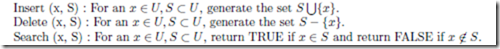Remark : When the universal set is evident in a context, we will not explicitly mention it in the discussions. Notice that we work with sets and not multisets. Thus, Insert (x,S) does not produce new set when x is in the set already. Similarly Delete (x, S) does not produce a new set when x /∈ S.

Due to its fundamental importance in a host of applications ranging from compiler design to data bases, extensive studies have been done in the design of data structures for dictionaries.

Refer to Chapters 3 and 10 for data structures for dictionaries designed with the worst-case costs in mind, and Chapter 12 of this handbook for a data structure designed with amortized cost in mind. In Chapter 15 of this book, you will ﬁnd an account of B-Trees which aim to minimize the disk access. All these structures, however, are deterministic. In this sequel, we discuss two of the interesting randomized data structures for Dictionaries. Speciﬁcally

• We describe a data structure called Skip Lists and present a comprehensive probabilistic analysis of its performance.

• We discuss an interesting randomized variation of a search tree called Randomized Binary Search Tree and compare and contrast the same with other competing structures.

## Preliminaries

In this section we collect some basic deﬁnitions, concepts and the results on randomized computations and probability theory. We have collected only the materials needed for the topics discussed in this chapter. For a more comprehensive treatment of randomized algorithms, refer to the book by Motwani and Raghavan .

Randomized Algorithms

Every computational step in an execution of a deterministic algorithm is uniquely deter- mined by the set of all steps executed prior to this step. However, in a randomized algorithm, the choice of the next step may not be entirely determined by steps executed previously; the choice of next step might depend on the outcome of a random number generator. Thus, several execution sequences are possible even for the same input. Speciﬁcally, when a ran- domized algorithm is executed several times, even on the same input, the running time may vary from one execution to another. In fact, the running time is a random variable depending on the random choices made during the execution of the algorithm. When the running time of an algorithm is a random variable, the traditional worst case complexity measure becomes inappropriate. In fact, the quality of a randomized algorithm is judged from the statistical properties of the random variable representing the running time. Speciﬁcally, we might ask for bounds for the expected running time and bounds beyond which the running time may exceed only with negligible probability. In other words, for the randomized algorithms, there is no bad input; we may perhaps have an unlucky execution.

The type of randomized algorithms that we discuss in this chapter is called Las Vegas type algorithms. A Las Vegas algorithm always terminates with a correct answer although the running time may be a random variable exhibiting wide variations. There is another important class of randomized algorithms, called Monte Carlo algorithms, which have ﬁxed running time but the output may be erroneous. We will not deal with Monte Carlo algorithms as they are not really appropriate for basic building blocks such as data structures.

We shall now deﬁne the notion of eﬃciency and complexity measures for Las Vegas type randomized algorithms.

Since the running time of a Las Vegas randomized algorithm on any given input is a random variable, besides determining the expected running time it is desirable to show that the running time does not exceed certain threshold value with very high probability. Such threshold values are called high probability bounds or high conﬁdence bounds. As is customary in algorithmics, we express the estimation of the expected bound or the high- probability bound as a function of the size of the input. We interpret an execution of a Las Vegas algorithm as a failure if the running time of the execution exceeds the expected running time or the high-conﬁdence bound.

DEFINITION 13.2 [Conﬁdence Bounds] Let α, β and c be positive constants. A ran- domized algorithm A requires resource bound f (n) with

1. nexponential probability or very high probability, if for any input of size n, the amount of the resource used by A is at most αf (n) with probability 1 O(βn), β > 1. In this case f (n) is called a very high conﬁdence bound.

2. n polynomial probability or high probability, if for any input of size n, the amount of the resource used by A is at most αf (n) with probability 1 O(nc ). In this case f (n) is called a high conﬁdence bound.

3. n log probability or very good probability, if for any input of size n, the amount of the resource used by A is at most αf (n) with probability 1 O((log n)c). In this case f (n) is called a very good conﬁdence bound.

4. high constant probability, if for any input of size n, the amount of the resource used by A is at most αf (n) with probability 1 O(βα ), β > 1.

The practical signiﬁcance of this deﬁnition can be understood from the following discussions. For instance, let A be a Las Vegas type algorithm with f (n) as a high conﬁdence bound for its running time. As noted before, the actual running time T (n) may vary from one execution to another but the deﬁnition above implies that, for any execution, on any input, P r(T (n) > f (n)) = O(nc). Even for modest values of n and c, this bound implies an extreme rarity of failure. For instance, if n = 1000 and c = 4, we may conclude that the chance that the running time of the algorithm A exceeding the threshold value is one in zillion.

Basics of Probability Theory

We assume that the reader is familiar with basic notions such as sample space, event and basic axioms of probability. We denote as P r(E) the probability of the event E. Several results follow immediately from the basic axioms, and some of them are listed in Lemma 13.1.

LEMMA 13.1 The following laws of probability must hold: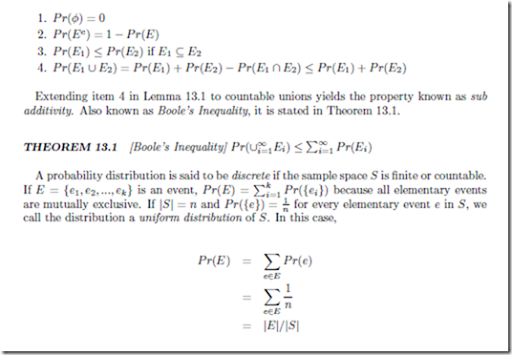which agrees with our intuitive and a well-known deﬁnition that probability is the ratio of the favorable number of cases to the total number of cases, when all the elementary events are equally likely to occur.

Conditional Probability

In several situations, the occurrence of an event may change the uncertainties in the oc- currence of other events. For instance, insurance companies charge higher rates to various categories of drivers, such as those who have been involved in traﬃc accidents, because the probabilities of these drivers ﬁling a claim is altered based on these additional factors.

DEFINITION 13.3 [Conditional Probability] The conditional probability of an event E1 given that another event E2 has occurred is deﬁned by P r(E1 /E2) (“P r(E1/E2)” is read as “the probability of E1 given E2.”).

LEMMA 13.2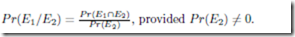Lemma 13.2 shows that the conditional probability of two events is easy to compute. When two or more events do not inﬂuence each other, they are said to be independent. There are several notions of independence when more than two events are involved. Formally,

DEFINITION 13.4 [Independence of two events] Two events are independent if P r(E1 E2) = P r(E1 )P r(E2 ), or equivalently, P r(E1/E2) = P r(E1).

DEFINITION 13.5 [Pairwise independence] Events E1, E2,... Ek are said to be pairwise independent if P r(Ei Ej ) = P r(Ei)P r(Ej ), 1 i /= j n.

Given a partition S1,... , Sk of the sample space S, the probability of an event E may be expressed in terms of mutually exclusive events by using conditional probabilities. This is known as the law of total probability in the conditional form.

LEMMA 13.3 [Law of total probability in the conditional form] For any partition S1, …, Sk of the sample space S, P r(E) = ),k P r(E/Si) P r(Si).

The law of total probability in the conditional form is an extremely useful tool for calculating the probabilities of events. In general, to calculate the probability of a complex event E, we may attempt to ﬁnd a partition S1, S2,…, Sk of S such that both P r(E/Si) and P r(Si) are easy to calculate and then apply Lemma 13.3. Another important tool is Bayes’ Rule.

THEOREM 13.2 [Bayes’ Rule] For events with non-zero probabilities,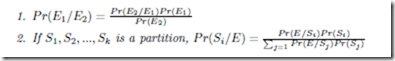Proof Part (1) is immediate by applying the deﬁnition of conditional probability; Part (2) is immediate from Lemma 13.3.

Random Variables and Expectation

Most of the random phenomena are so complex that it is very diﬃcult to obtain detailed information about the outcome. Thus, we typically study one or two numerical parameters that we associate with the outcomes. In other words, we focus our attention on certain real-valued functions deﬁned on the sample space.

DEFINITION 13.6 A random variable is a function from a sample space into the set of real numbers. For a random variable X, R(X) denotes the range of the function X.

Having deﬁned a random variable over a sample space, an event of interest may be studied through the values taken by the random variables on the outcomes belonging to the event. In order to facilitate such a study, we supplement the deﬁnition of a random variable by specifying how the probability is assigned to (or distributed over) the values that the random variable may assume. Although a rigorous study of random variables will require a more subtle deﬁnition, we restrict our attention to the following simpler deﬁnitions that are suﬃcient for our purposes.

A random variable X is a discrete random variable if its range R(X) is a ﬁnite or countable set (of real numbers). This immediately implies that any random variable that is deﬁned over a ﬁnite or countable sample space is necessarily discrete. However, discrete random variables may also be deﬁned on uncountable sample spaces. For a random variable X, we deﬁne its probability mass function (pmf ) as follows:

DEFINITION 13.7 [Probability mass function] For a random variable X, the probability mass function p(x) is deﬁned as p(x) = P r(X = x), x R(X).

The probability mass function is also known as the probability density function. Certain trivial properties are immediate, and are given in Lemma 13.4.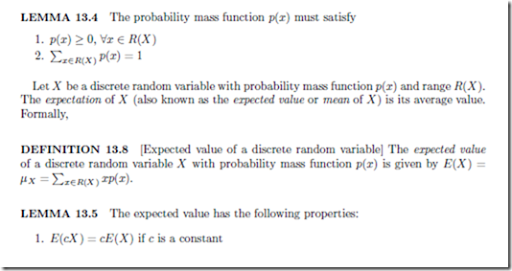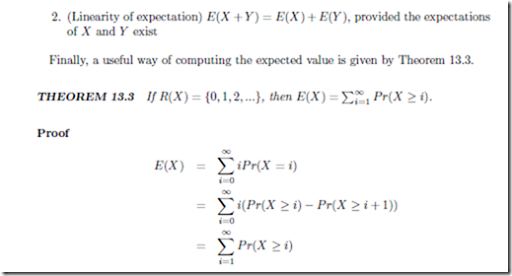Some Basic Distributions

Bernoulli Distribution

We have seen that a coin ﬂip is an example of a random experiment and it has two possible outcomes, called success and failure. Assume that success occurs with probability p and that failure occurs with probability q = 1 p. Such a coin is called p-biased coin. A

coin ﬂip is also known as Bernoulli Trial, in honor of the mathematician who investigated extensively the distributions that arise in a sequence of coin ﬂips.

DEFINITION 13.9 A random variable X with range R(X) = {0, 1} and probability mass function P r(X = 1) = p, P r(X = 0) = 1 p is said to follow the Bernoulli Distribution. We also say that X is a Bernoulli random variable with parameter p.

Binomial Distribution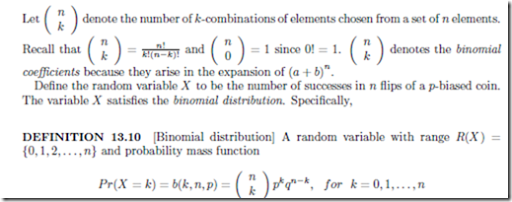THEOREM 13.4 For a binomial random variable X, with parameters n and p, E(X) = np and V ar(X) = npq.

Geometric Distribution

Let X be a random variable X denoting the number of times we toss a p-biased coin until we get a success. Then, X satisﬁes the geometric distribution. Speciﬁcally,

DEFINITION 13.11 [Geometric distribution] A random variable with range R(X) = {1, 2,... , } and probability mass function P r(X = k) = qk1p, for k = 1, 2,... , satisﬁes the geometric distribution. We also say that X is a geometric random variable with parameter p.

The probability mass function is based on k 1 failures followed by a success in a sequence of k independent trials. The mean and variance of a geometric distribution are easy to compute.

Negative Binomial distribution

Fix an integer n and deﬁne a random variable X denoting the number of ﬂips of a p– biased coin to obtain n successes. The variable X satisﬁes a negative binomial distribution. Speciﬁcally,

DEFINITION 13.12 A random variable X with R(X) = {0, 1, 2, …} and probability mass function deﬁned by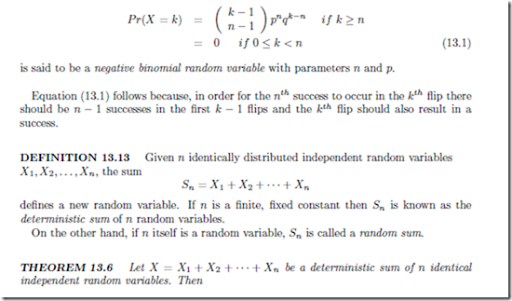1. If Xi is a Bernoulli random variable with parameter p then X is a binomial random variable with parameters n and p.

2. If Xi is a geometric random variable with parameter p, then X is a negative binomial with parameters n and p.

3. If Xi is a (negative) binomial random variable with parameters r and p then X is a (negative) binomial random variable with parameters nr and p.

Deterministic sums and random sums may have entirely diﬀerent characteristics as the following theorem shows.

THEOREM 13.7 Let X = X1 + ··· + XN be a random sum of N geometric random variables with parameter p. Let N be a geometric random variable with parameter α. Then X is a geometric random variable with parameter αp.

Tail Estimates

Recall that the running time of a Las Vegas type randomized algorithm is a random variable and thus we are interested in the probability of the running time exceeding a certain threshold value.

Typically we would like this probability to be very small so that the threshold value may be taken as the ﬁgure of merit with high degree of conﬁdence. Thus we often compute or estimate quantities of the form P r(X k) or P r(X k) during the analysis of randomized algorithms. Estimates for the quantities of the form P r(X k) are known as tail estimates.

The next two theorems state some very useful tail estimates derived by Chernoﬀ. These bounds are popularly known as Chernoﬀ bounds. For simple and elegant proofs of these and other related bounds you may refer .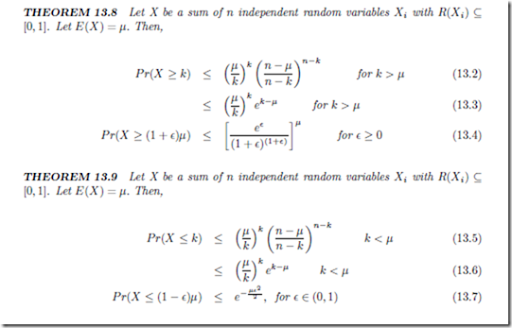Recall that a deterministic sum of several geometric variables results in a negative binomial random variable. Hence, intuitively, we may note that only the upper tail is meaningful for this distribution. The following well-known result relates the upper tail value of a negative binomial distribution to a lower tail value of a suitably deﬁned binomial distribution. Hence all the results derived for lower tail estimates of the binomial distribution can be used to derive upper tail estimates for negative binomial distribution. This is a very important result because ﬁnding bounds for the right tail of a negative binomial distribution directly from its deﬁnition is very diﬃcult.

THEOREM 13.10 Let X be a negative binomial random variable with parameters r and p. Then, P r(X > n) = P r(Y < r) where Y is a binomial random variable with parameters n and p.